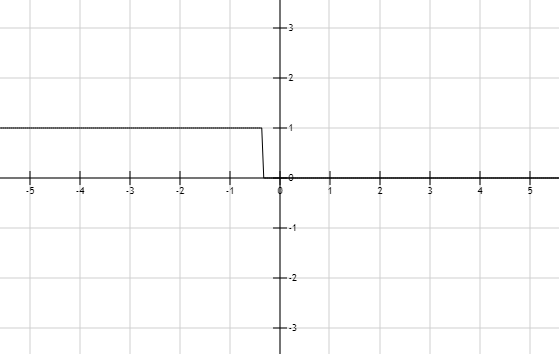# The solution of given linear inequality equation and plot a graph for it.### Precalculus: Mathematics for Calcu...

6th Edition
Stewart + 5 others
Publisher: Cengage Learning
ISBN: 9780840068071### Precalculus: Mathematics for Calcu...

6th Edition
Stewart + 5 others
Publisher: Cengage Learning
ISBN: 9780840068071

#### Solutions

Chapter 1.7, Problem 22E
To determine

## The solution of given linear inequality equation and plot a graph for it.

Expert Solution

x<0.33

### Explanation of Solution

Given:

Linear inequality equation

2x5+1<152x

Calculation,

Linear inequality equation,

2x5+1<152x2x5+2x<1512x+10x5<15512x5<4512x<4x<412x<0.33

Now, graph of 2x5+1<152xConclusion:

Hence, the values of x is less than the 0.33.

### Have a homework question?

Subscribe to bartleby learn! Ask subject matter experts 30 homework questions each month. Plus, you’ll have access to millions of step-by-step textbook answers!